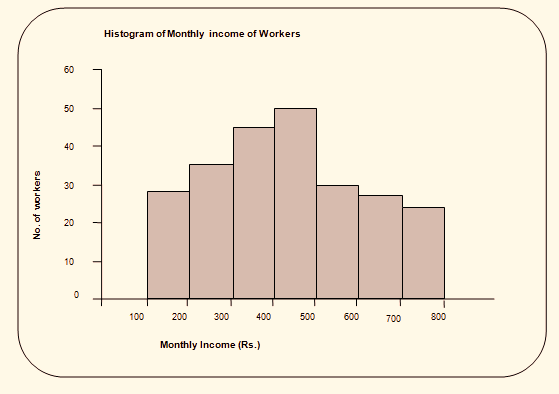# Histogram - Diagrammatic and Graphical Representation of Data | Economics Notes Grade XI

### Commerce Notes

☰   Related Articles

#### Scarcity And Choice

Concepts of Scarcity And Choice

#### Extra# Histogram - Diagrammatic and Graphical Representation of Data

The histogram is the graphical presentation of the continuous frequency distribution drawn by the help of rectangular vertical bars whose heights represent the frequencies of the classes. The width of the bar remains the same if the class interval is equal and the width may vary in case of unequal class intervals. In histograms, the class intervals are measured along the x-axis and frequencies along the y-axis. While constructing a histogram, the following considerations should be made.

• For a histogram, the frequency distribution should be continuous and exclusive.
• Mid-points given: If only the mid-points are given, the distribution must be converted into a continuous one in the exclusive series.
• Discrete frequency distribution: Histogram can be used to present discrete frequency distribution by converting the discrete values into continuous series.
• Open-end classes: In the case of open-end classes, the histogram cannot be constructed. But if a histogram is to be drawn, the class interval is assumed to be the same at the end class interval as in other classes.
• Histogram with unequal classes: In the case of the unequal class of frequency distribution, the class interval must be equal before constructing the histogram.

Example:

 Monthly income (Rs.) (No. of workers) 100 – 200 28 200 – 300 35 300 – 400 45 400 – 500 50 500 – 600 30 600 – 700 27 700 – 800 24Above diagram is the example of Histogram.

## Popular Subjects

Join with us on social media to see our updates on your feed.